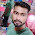### Program 231: Decryption of Viginere Cipher using C

Program 231:

```#include<stdio.h>
#include<string.h>
#include<ctype.h>
#include<stdlib.h>
main()
{
int i,j,k,numstr,numkey,numcipher;
char str,key;
printf("Enter a string to Decrypt\n");
gets(str);
//converting entered string to Capital letters
for(i=0,j=0;i<strlen(str);i++)
{
if(str[i]!=' ')
{
str[j]=toupper(str[i]);
j++;
}
}
str[j]='\0';
printf("Entered string is : %s \n",str);
//Storing string in terms of ascii
for(i=0;i<strlen(str);i++)
{
numstr[i]=str[i]-'A';
}
printf("Enter a key\n");
gets(key);
//converting entered key to Capital letters
for(i=0,j=0;i<strlen(key);i++)
{
if(key[i]!=' ')
{
key[j]=toupper(key[i]);
j++;
}
}
key[j]='\0';
//Assigning key to the string
for(i=0;i<strlen(str);)
{
for(j=0;(j<strlen(key))&&(i<strlen(str));j++)
{
numkey[i]=key[j]-'A';
i++;
}

}

for(i=0;i<strlen(str);i++)
{
numcipher[i]=numstr[i]-numkey[i];//changed from + to - for decryption
if(numcipher[i]<0)
{
numcipher[i]+=26;
}
}

printf("Decrypted Vigenere Cipher text is\n");
for(i=0;i<strlen(str);i++)
{
printf("%c",(numcipher[i]+'A'));
}

printf("\n");
}```
Explanation:

//Coming Soon
Output:

#### 1 comment:

1.factorial hundred In the last few days, the “factorial of 100” is one of the top subjects and a lot of maths geeks compute it using voice assistants such as Alexa, Shiri, etc.

factorial hundred In the last few days, the “factorial of 100” is one of the top subjects and a lot of maths geeks compute it using voice assistants such as Alexa, Shiri, etc.

factorial hundred In the last few days, the “factorial of 100” is one of the top subjects and a lot of maths geeks compute it using voice assistants such as Alexa, Shiri, etc.

vegamovies Earlier people were not so much means to watch movies and to watch movies, we had to go to the movies hall where we were not allowed to go without tickets, but now people can watch movies online since the internet became cheaper.

 Donate Buy me a coffee \$2.00 USD Buy me a burger \$5.00 USD Buy me a pizza \$10.00 USDCompiler Used by me C-Free 5.0(Recommended) Other Compilers Code Blocks(Recommended) Online Compilers Ideone(Remember to give input before executing online where ever necessary)